#Newton's Law of CoolingFrom experimental observations it is known that (up to a ``satisfactory'' approximation) the surface temperature of an object changes at a rate proportional to its relative temperature. That is, the difference between its temperature and the temperature of the surrounding environment. This is what is known as Newton's law of cooling. Thus, if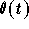is the temperature of the object at time t, then we have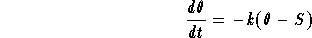where S is the temperature of the surrounding environment. A qualitative study of this phenomena will show that k >0. This is a first order linear differential equation. The solution, under the initial condition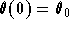, is given by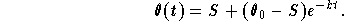Hence,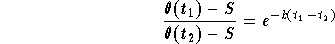,

which implies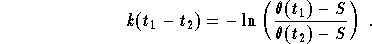This equation makes it possible to find k if the interval of time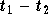is known and vice-versa.

Example: Time of Death Suppose that a corpse was discovered in a motel room at midnight and its temperature was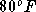. The temperature of the room is kept constant at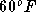. Two hours later the temperature of the corpse dropped to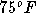. Find the time of death.

Solution: First we use the observed temperatures of the corpse to find the constant k. We have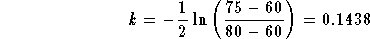.

In order to find the time of death we need to remember that the temperature of a corpse at time of death is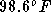(assuming the dead person was not sick!). Then we have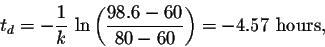which means that the death happened around 7:26 P.M.

One of our interested readers, E.P. Esterle, wrote a program that helps find the time of death based on the above notes. Click HERE to download it. Have fun with it.[Differential Equations] [Separable Equations]
[Geometry] [Algebra] [Trigonometry ]
[Calculus] [Complex Variables] [Matrix Algebra]S.O.S MATHematics home page

Do you need more help? Please post your question on our S.O.S. Mathematics CyberBoard.Author: Mohamed Amine Khamsi